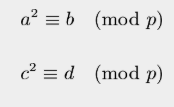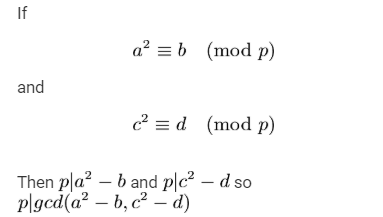# Guess The Prime

In the July Challenge Guess the prime who will set the prime number

And who will give the output of x^2%P

You may refer to this

Thank you.

2 Likes

Lol, the test cases were really weak.
I just entered two numbers such that -And took the largest prime factor of gcd(a^2 - b , c^2 - d).

After tinkering with several a,c I got AC

This is a very common verdict ig.

I was getting it initially.
Then I got a verdict where opposite cases were AC and there was a TLE -1.00000 in some cases.

So, I multiplied my numbers and got AC 100pts

I took two perfect squares. Took the mod answer from judge and subtracted to the square to make it a multiple.

Computed GCD of both the numbers and did its prime factorization. Took the largest prime factor which was the answer.

And why this is working?

Did you get it in first try?
Coz not all squares were giving AC

I had same logic btw but did a lot aof submits to find numbers which gave ACYes, I used the same logic. Used a page for proof lol.

Started with a large enough number (x = 31623 I think), computed the factorization of (x^2-r) => these are all the possible candidates. Then I tried numbers y > x until I found one for which all of the candidates give a different rest.

1 Like